The first goal in any high-speed board stack up design is to engineer interconnects with a target impedance, and the first step in this process is to use a 2D field solver to explore design space with a virtual prototype. Just how well can a field solver predict the impedance of traces on a real board?

For various projects at the University of Colorado Boulder, we built boards from different vendors and found the measured impedance of traces different from what we expected based on the dimensions and Dk values provided. The difference was more than 15% in a few cases. For some test vehicles that we wanted to use to illustrate signal integrity principles, we needed to engineer specific impedance traces much more accurately. This requirement prompted this study.

Our plan was to use a 2D field solver to accurately predict the impedance of fabricated traces on circuit boards. However, to do this, we needed an accurate value of the dielectric constant, Dk, of the layers. Using an on-board test structure to extract the Dk of the fabricated board, we found that we can routinely achieve trace impedances within less than 3% of our target value. Here is how we did it.

### A Test Vehicle

For this experiment, we used the Polar Instruments SI9000 tool as the 2D field solver. The first step was to evaluate the relative accuracy of this tool against other 2D tools. (This was done in .) The simulated impedance for the same geometry differential pair transmission lines was found to agree to within 1% for all commercial tools evaluated.

But in practice, how well will the agreement between the prediction of the 2D field solver match the impedance of traces on a fabricated board?

To quickly explore this question, we used an LPKF milling machine to mill out various width uniform transmission lines on a 59 mil thick copper clad circuit board, including the outer copper layers (see Figure 1). Each trace was six inches long. The bottom layer (not visible) was a solid, continuous ground plane.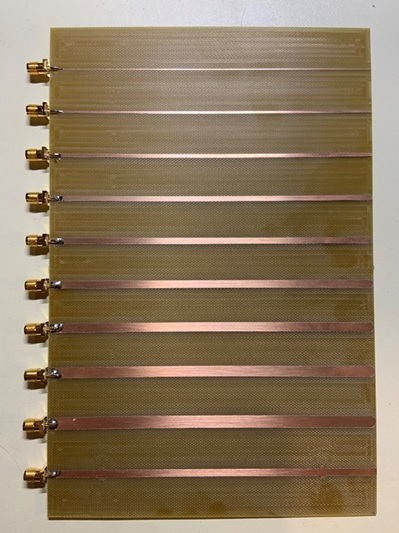Figure 1. Top surface of the test board with different line width traces. The traces are 6 inches long.

After an SMA connector was soldered to the edge of each trace, we measured the TDR response using a Teledyne Test Tools T3SP15D TDR with a 50 psec rise time. The TDR profiles show the uniformity of the instantaneous impedance of each trace. The TDR absolute accuracy has been confirmed to better than 1% accuracy .

This measured data shown in Figure 2 clearly shows the trend of wider lines having lower characteristic impedance. In addition, we see the round-trip time delay increase for the wider and lower impedance traces. This is because the effective Dk of these traces increases for wider lines. The narrowest lines show the most impedance variation due to slight line width variations from the milling process.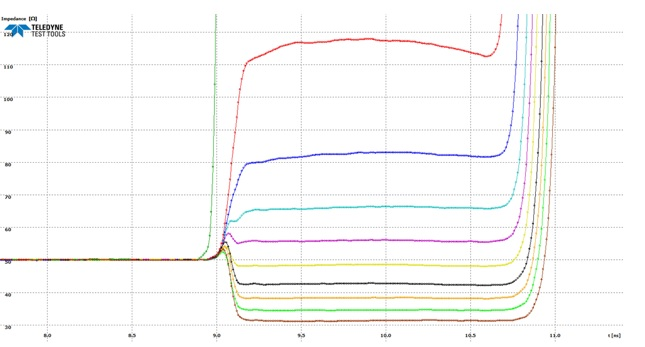Figure 2. Measured TDR profiles of each line with an SMA connector.

In order to compare these measured impedances with the predictions from the 2D field solver, we need to provide it with four inputs:
• Dielectric thickness
• Copper thickness
• Line width
• Dielectric constant

The dielectric thickness was measured as 56.2 mils ±0.5 mils. The copper thickness on both surfaces was 1 oz copper which is assumed to be 1.4 mils thick. The line widths were measured with an optical microscope with an absolute accuracy to within 3%. The last remaining term we needed to input into the 2D field solver was the dielectric constant of the board. When asked, the board vendor said it is 4.2, and all they offered was one value and no idea of the frequency. But is it really Dk = 4.2?

### Measuring the Dk

A vendor typically provides a stack up drawing for the dimensions of each layer. Sometimes they do not provide the value of the Dk of each layer, or even worse, sometimes they provide a value, but it turns out to be wrong.

In a previous study, we developed a simple test pattern that is placed on all our boards to measure the Dk of a specific laminate layer. This is a uniform transmission line with two small discontinuities a precision distance apart.

In a TDR measurement of the trace, the round-trip time delay between the two discontinuities is measured to within 10 psec. From the time delay and length, the signal velocity is calculated, and, from this, the effective Dk. Figure 3 shows a closeup of the small pad that causes the first reflection.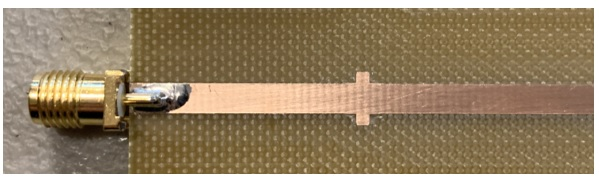Figure 3. Close up of the uniform transmission line with a small capacitive discontinuity providing a reference mark in the TDR response.

The measured TDR response from this test line is shown in Figure 4. The round-trip time delay between the dips is measured as 1.24 ±0.01 nsec, so the one-way time delay is 0.62 nsec. The effective Dk is calculated as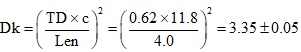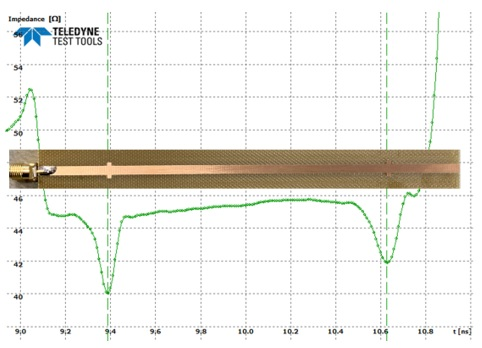Figure 4. Measured TDR response of the test line on the board with two small discontinuities spaced 4.00 inches apart. The variation in impedance along the line is due to the slight line width variation from the milling process.

What we measure is the effective Dk. What we need is the bulk Dk. We have to use a 2D field solver to back out a value of the bulk Dk that results in a calculated effective Dk matching our measurement. This is done in just a few iterations of the field solver. The microstrip dimensions of the test line were:

H1 = 56.2 mils
W1 = W2 = 125 mils
T1 = 1.4 mils

We adjusted the Er1 value in the SI9000 tool until the effective Dk calculated matched the measured value of 3.35. The final value of bulk Er1 (which gave an effective Dk value of 3.349 is 4.39) is shown in Figure 5.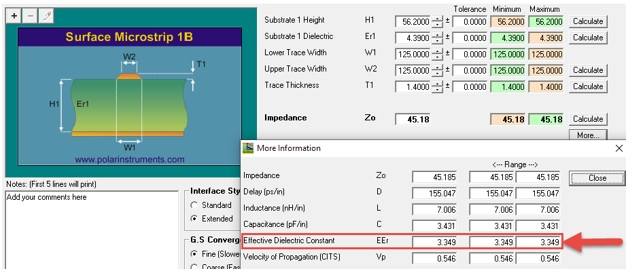Figure 5. We back out the bulk dielectric constant as the value that gives the effective Dk equal to the measurement, highlighted in red.

The vendor supplied value was 4.2. This is 5% different, in this example. The calculated characteristic impedance for this line is 45.18 ohms. The measured value from the TDR is 45.5 ±0.5 ohms, within the roughly 1% uncertainty in the uniformity of the impedance profile.

### Comparing “as Designed” and “as Measured” Impedances

The measured impedance of each line on the board was compared to the calculated value for each line, given the dimensions and the measured bulk Dk of 4.39. This comparison is shown in Figure 6.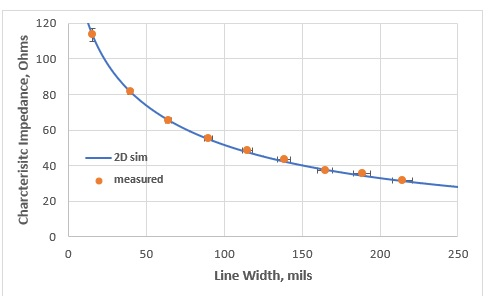Figure 6. Comparing the measured and predicted values of characteristic impedance based on the measured Dk.

Over this range, the worst-case difference was 2.9%. This is the within the absolute accuracy of the line width dimensions.

In this board, the basic uncertainty was in the linewidth, limited by our optical measurement system. Once the Dk was measured, it was possible to predict the characteristic impedance of an as fabricated line to better than 3%.

It is always an important confidence builder when we see a measured value match a simulated value to within the accuracy of the measurement. It doesn’t mean the simulation is correct, it just means that the measurements and the predictions from the 2D field solver are consistent.

This simple experiment demonstrates that with good input information, a 2D field solver can be an accurate predictor of performance.

### Designed Target Impedance

In our lab, we routinely add a test line to all boards to measure the Dk of the important layers. Once we are familiar with a specific stack-up with a specific vendor, we stay with that stack up. We can track the stability of the vendor’s process with each board build.

Figure 7 is an example of a test line with two small discontinuities spaced exactly 2.0 inches apart and its TDR profile. The measured round-trip delay between the reflections is 0.635 ±0.01 nsec. The effective Dk is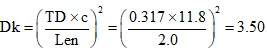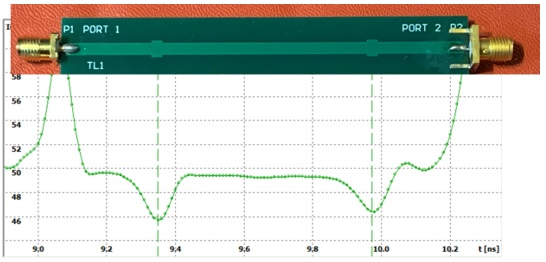Figure 7. A test line on each board to measure the Dk and confirm the Z0 with the measured TDR profile for this line.

Using the same process as before, the bulk Dk that we back out for this board is 4.63. This assumes a 1 mil thick soldermask on the top surface. Because of the wide conductor, its impact reduces the impedance by only 0.6% and increases the Dk value by 1%.

The vendor specified Dk of the core was 3.96. This is more than a 17% difference from what we measured.  Because we had run previous  boards through this vendor’s shop, we knew what the bulk Dk was and designed our test lines using our measured value and not the vendor’s.

Using the bulk Dk of 4.63, the dielectric thickness of the layer and the 2D field solver, we can engineer the line width to achieve a target impedance of 50 Ohms. The measured impedance of this fabricated trace is seen to be 49.5 Ohms, within 1% of the target value.  Using this approach, we can engineer any impedance value we need using the 2D field solver.

### Conclusion

It is possible to engineer fabricated circuit board traces with target impedances within 1% of the designed impedance using a combination of an accurate 2D field solver, a fab vendor with a stable process, and a special test trace on each board to measure and track the bulk dielectric constant of each layer.

For routine high-speed applications, this level of accuracy is not needed. But, for special test boards that demonstrate specific signal integrity principles, this technique is very important to create predictable interconnect structures.

When the dielectric layers are thinner, and line widths are narrower, the impact of the soldermask plays a slightly larger role. In this case, fabricating a board with test traces having no soldermask will enable better accuracy in the extracted Dk.

While this method was applied to simple 2-layer boards in this example, it can be scaled to multilayer boards as well, where the Dk of each layer should be measured to achieve the desired target impedance.

### References

1. Narula, Gaurav, and Eric Bogatin. “Field Solvers for Transmission Line Analysis: How Similar Are They?” Printed Circuit Design & Fab Online Magazine - Field Solvers for Transmission Line Analysis: How Similar Are They?, www.pcdandf.com/pcdesign/index.php/editorial/menu-features/10472-simulation-1512.

2. Bogatin, Eric. “Test Your TDR with a DMM.” Signal Integrity Journal , Signal Integrity Journal, 10 June 2019, www.signalintegrityjournal.com/blogs/8-for-good-measure/post/1282-test-your-tdr-with-a-dmm.